• 平面Σ与三个坐标轴的交点分别为 A(a,0,0),B(0,b,0),C(0,0,c),其中a，b，c为非零常数，则平面∑的方程为 ∑：x/a + y/b + z/c = 1 推导： 根据A、B、C三点可得向量AB(-a,b,0)和向量AC(-a,0,c)，求得这两个向量的...
背景与结论如下： 设平面Σ与三个坐标轴的交点分别为 A(a,0,0),B(0,b,0),C(0,0,c),其中a，b，c为非零常数，则平面∑的方程为 ∑：x/a + y/b + z/c = 1
推导： 根据A、B、C三点可得向量AB(-a,b,0)和向量AC(-a,0,c)，求得这两个向量的向量积为向量n-(bc,ac,ab)根据平面点法式方程的定义，将向量n和A点代入：展开全文• 平面方程有四种表达方式分别是：截距式，点法式，一般式，法线式。 1.点法式 A(x-x0)+B(y-y0)+C(z-z0)=0 假设n=(A,B,C)为平面的法向量，M=(x,y,z)为平面上任意一点，M'=(x0,y0,z0)，则有n·MM'=0,则有A(x-x0)+B(y...
平面方程有四种表达方式分别是：截距式，点法式，一般式，法线式。
1.点法式
A(x-x0)+B(y-y0)+C(z-z0)=0
假设n=(A,B,C)为平面的法向量，M=(x,y,z)为平面上任意一点，M'=(x0,y0,z0)，则有n·MM'=0,则有A(x-x0)+B(y-y0)+C(z-z0)=0
2.一般式
Ax+By+Cz+D=0
由点法式推出A*x + B*y + C*z - A*x0 - B*y0 - C*z0 = 0 令D =  - A*x0 - B*y0 - C*z0即推导出一般式
3.截距式
设平面方程为Ax+By+Cz+D=0,若D不等于0，取a=-D/A,b=-D/B,c=-D/C,则得平面的截距式方程：x/a + y/b + c/z = 1
平面与三个轴的坐标分别为P(a,0,0),Q(0,b,0),R(0,0,c)，其中，a,b,c依次称为该平面在x,y,z轴上的解距。
推导过程很简单平面与x轴的交点就是令y=0,z=0,所以a= -D/A，以此类推出b=-D/B,c=-D/C。
4.法线式
xcosα + ycosβ + zcosγ = d 其中cosα、cosβ、cosγ是平面法向量的方向余玹，p为原点到平面的距离。其中cosα²＋cosβ²＋cosγ²＝１
假设原点到平面的法线为on，平面上任意一点p(x,y,z)，平面上一点p₀,(x₀,y₀,z₀)，则有on·pp₀ = 0。
pp₀ = op - op₀，则on·op - on·op₀ = 0
假设on₀的的单位法向量为（cosα，cosβ，cosγ）且 cosα²＋cosβ²＋cosγ²＝１则on·op - on·op₀ = on₀·op - on₀·op₀
其中on₀·op₀ = d 并且 on₀·op = xcosα + ycosβ + zcosγ 所以xcosα + ycosβ + zcosγ = d


展开全文• 平面方程的基本定义 三维空间中所有处于同一平面的点所对应的方程，任何一个平面都可以用三元一次方程来表示。 确定一个平面需要的条件 点法式： 1、平面上的一个点； 2、以该点为起点的法向量。 标准： ...
平面方程的基本定义
三维空间中所有处于同一平面的点所对应的方程，任何一个平面都可以用三元一次方程来表示。
平面方程表达式
表达式限定条件特殊含义一般式Ax+By+Cz+D=0A,B,C,D为已知常数，并且A,B,C不同时为零。 D=0时，该平面过原点， (A,B,C)是该平面的法向量。 点法式A(x-x0)+B(y-y0)+C(z-z0)=0A,B,C,D为已知常数，并且A,B,C不同时为零。(A,B,C)是该平面的法向量，(x0,y0,z0)是该平面上的点。截距式x/a+y/b+z/c=1若该平面在一个轴上没有截距，则这个平面平行于该轴，表达式为两元一次方程；若该平面在两个轴上没有截距，则这个平面平行于这两个轴，表达式为一元一次方程。该平面过(a,0,0),(0,b,0),(0,0,c)，a,b,c依次称为该平面在x,y,z轴上的截距。法线式xcosα+ycosβ+zcosγ=p p为原点到平面的距离，cosα、cosβ、cosγ是平面法向量的方向余弦。。

确定一个平面需要的条件
点法式：
1、平面上的一个点；
2、该平面的法向量。

标准式：
1、平面上的一个点；
2、两个不共线且与平面平行的向量。

三点式：
1、三个不同时共线的点。

注意：若已知三个点求平面方程，通常利用这三个点得出两个向量，然后转化为点法式或标准式。
推导过程
推导（因为输入法不便，向量上方箭头省略。）备注点法式 已知：一个平面过点M0(x0,y0,z0)，平面的法向量为n(A,B,C)，其中A、B、C不同时为0。 证明：取平面内的一个点M(x,y,z),则n⊥MM0，即向量MM0(x-x0,y-y0,z-z0)与n内积等于0。 ⇒A(x-x0)+B(y-y0)+C(z-z0)=0。  一般式三维空间中所有处于同一平面的点所对应的方程，任何一个平面都可以用三元一次方程来表示。 截距式 已知：一个平面过(a,0,0),(0,b,0),(0,0,c)三点。 证明：设这个平面的方程为Ax+By+Cz+D=0，将三个点带入后得到A=-D/a,B=-D/b,C=-D/c，将ABC三个值分别带入到方程中，通过移项整理后，⇒x/a+y/b+z/c=1  法线式略。


展开全文• 点到面的距离一般还说时最短距离，但一个平面一般是有界限的，所以需要先把一个平面截距式方程显示为： Ax+By+Cz+D=0 借用这张图来表示： 可以看到要求d（最短距离）需要知道q点到p点（p点时平面的点）的距离...
前几天跟同事讨论一个几何知识时遇到了一些疑问，后面经过推导弄明白了，其实这道题不难，但是里面有些基本的原理深究的话一开始还是没想明白，在这里记录下：
点到面的距离一般还说时最短距离，但一个平面一般是有界限的，所以需要先把一个平面用截距式方程显示为：
Ax+By+Cz+D=0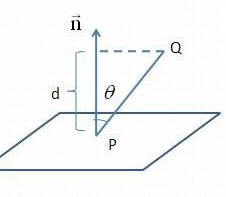借用这张图来表示：
可以看到要求d（最短距离）需要知道q点到p点（p点时平面的点）的距离（也就是他的模），以及这个角度，
通过三角函数知道：d=
因为pg的模本身我们不确定，所以需要得到一个确定的值来运算
我们可以用来做运算，要得到这块我们法线d这个公式只需要加上就可以了，
所以我们乘除得到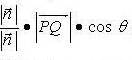已知=
得：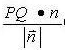到了这一步我们法线我们得法向量还未知：
但其实我们通过截距式方程的公式可知n={A,B,C}。
当然我们还时可以推导出来的。
法向量的推导可以用两个方式，一个是取平面的任意两条线与法向量点乘，这个值必然为0。另一个是用任意两条平面的线做叉乘，这时可得垂直于他的法向量。
下面我们推导点乘的方式：
我们已知平面的截距式公式为：Ax+By+Cz+D=0
得到：截距式表明了，当y=0，z=0时，跟x轴相交，代入公式得到,
同理y=0，z=0时，跟y轴相交，带入公式得到y=
同理x=0，y=0时，跟z轴相交，带入公式得到z=
然后我们可以得到平面的特殊的三个点a点：(,0,0)，b点：(0,,0),c点：(0,0,)
那么我们可以得到向量ab:,ac:,bc:
具体得到ab:(,,0),ac:(,0,),bc:(0,,)
我们之前说了平面和法向量的点乘一定为0
点乘的公式为：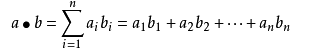带入进入得
*x+*y+0*z = 0
*x+0*y+*z=0
0*x+*y+*z=0
这里公式列出来我们可以求解，我们可以得到两种情况可以让xyz使上面得值为0得，
第一个值时xyz都为0，这个就i是具体的一个点了，而不是面。
那么另外一个值就只有点(A,B,C)了。
由此可得法向量为(A,B,C)

最终带入到上面的公式中得：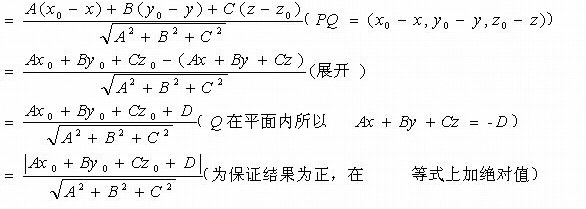（其中(x0,y0,z0)是点Q的值，ABCD是截距式公式的4个常量值）
最后的等式就是我们要求的点到面的最短距离了。
只需要带入具体的值就能算出来了。

这个公式在具体的应用中也会用到。

有时我们能直接套公式不需要推导就能完成很多事情，但只有完整推导出公式才属于自己的知识，才能在以后的复杂应用中灵活引用。
展开全文最短距离
• 平面的点法式方程 法向量：垂直于一个平面的非零向量叫做一个平面的法向量。 假设空间内有一点M0(x0,y0,z0)M0(x0,y0,z0)M_0(x_0, y_0, z_0)和一个向量n→=(A,B,C)n→=(A,B,C)\overrightarrow n = (A, B, C)，则...
• ## 点到平面距离推导

千次阅读 2018-10-12 09:54:13
截距式 x/a+y/b+z/c=1 点法式 A(x-x0)+B(y-y0)+C(z-z0)=0 一般式 Ax+By+Cz+D=0 法线式 xcosα+ycosβ+zcosγ=p 一、截距式平面方程为Ax+By+Cz+D=0,若D不等于0，取...
• 平面方程表示 “平面方程”是指空间中所有处于同一平面的点所对应的方程，其一般式形如Ax+By+Cz+D=0。 表示方法： ...截距式 x/a+y/b+z/c=1 点法式 A(x-x0)+B(y-y0)+C(z-z0)=0 一般式 Ax+By+Cz+D...数学
• 转自：http://www.cnblogs.com/graphics/archive/2010/07/10/1774809.html准备知识平面的一般式方程Ax +By +Cz + D = 0其中n = (A, B, C)是平面的法向量，D是将平面平移到坐标原点所需距离（所以D=0时，平面过原点）...
• 即: 分离超平面由法向量w和截距b（w, b）决定 该平面可以将特征空间分为两类 一部分为正类 一部分为父类 特殊情况: 当样本数据集为线性可分时 存在无穷多个可分割超平面 此时 引入概念 间隔最大化 来求最优...支持向量机
• 1. 平面截距式方程 2. 平面方程求解示例 3. 点到平面的距离公式的推导 4. 练习
• ## 点法式方程

千次阅读 2017-02-06 15:47:41
平面方程的三种表示法:点法式、一般式、截距式主要使用点法式方程：1. C++标准模板库从入门到精通 http://edu.csdn.net/course/detail/33242.跟老菜鸟学C++http://edu.csdn.net/course/detail/29013. 跟老菜鸟学...3d 图形
• ## 梯度下降法

千次阅读 2019-08-08 18:16:23
​ 在直线方程中，倒数代表斜率，在曲线方程中，倒数代表切线的斜率。倒数代表着参数theta单位变化时，损失函数J相应的的变化。通过上面图中的点可以发现，改点的导数为负值，所以随着参数theta的增加，损失函数J...梯度下降
• 线性方程的几何意义二元线性方程　该方程是一个二元线性方程组，包含两个方程，每个方程是一条直线，两条直线的交点就是该方程有唯一解...　方程x + 4y + z = 8，在xyz三个坐标轴上的截距分别是(8,0,0),(0,2,0),(0,0...直线 向量
• 一、法向量定义 定义：如果，那么向量叫做平面的法向量。平面的法向量共有两大类（从方向上分），无数条。...由，得·=0且·=0，由此得到关于x,y的方程组，解此方程组即可得到。 2、 任何一个x,y,z的一exception 图形 c
• 1-1 认识方程式 ...在座标平面一元一次方程式的图形是一条直线，上述的公式，我们也可以当作3是方程式的a，将-21当作是方程式的b。 1-3函数 现在将前一小节的公式近一步处理：1. 上述的0用y代替： ...数学
• SVM(支持向量机)最初是一种解决二分类的有监督学习算法，其目的在于：在给定两类样本的数据集的前提下，寻找一个将两类样本分隔开的超平面(separating hyperplane)，并且使得两类样本之间的边界间隔(margin)最大化。...支持向量机 分类算法 机器学习
• 本文从最简单一维样本情况引出线性回归的概念，进而使用中心极限定理说明样本点与回归函数值之间的...使用最大似然估计推导出最小二乘法，然后利用梯度为0求出最小二乘的驻点。最终给出线性回归参数$\theta$的解析线性回归 最小二乘法 机器学习 中心极限定理 最大似然估计
• 声明：本文是对西瓜书中第五章神经网络中公式推导过程补充，文章作为自己的学习笔记，欢迎大家于本人学习交流。未经本人许可，文章不得用于商业用途。转载请注明出处 本文章参考 作者： 周志华 书名：《机器学习》 ...机器学习 神经网络 算法
• 能够感觉到他对自己的要求也很高，也都是自己独立推导数学公式，优化算法，哈哈，以后又多了一个能够交流的小伙伴。然后也跟哈深的基友交换了看法，都是感觉自己能够看懂别人的算法，但是自己独立实现还是有困难，...支持向量机 svm
• 文章目录简述表示方法截距式点法式一般式法线式其他方式表示平面法线+空间内两点补充：表示三角面片的方法（区域） ...设平面方程为Ax+By+Cz+D=0,若D不等于0，取a=-D/A,b=-D/B,c=-D/C,则得平面截距式方程：x/a+...
• 文章目录简介引入怎么确定划分规则呢感知机数学原理ax+b和wx+b寻找损失函数间隔的度量函数间隔几何间隔点到超平面距离的证明损失函数梯度下降法进行优化 简介 本来应该在贝叶斯和SVM之前推出感知机的，但是感知机...机器学习 算法 感知机
• 内容来自百度百科知识以及东方耀老师笔记内容的整合 1、直线 1.1 定义 ...在平面上过不重合的两点有且只有一条直线，即不重合两点确定一条直线。在球面上，过两点可以做无数条类似直线。 1.
• 其中关于如何推导出最终的优化目标函数（见文末(2.14)(2.14)）主要有两种方式。第一种就是本文所介绍的，直接通过一个（几何）距离来推导，如周志华机器学习中的SVM就是采用的这种方式；第二种就是下文中所要介绍的...机器学习 svm
• 这是因为之前有个负号在方程外面，但是，这里我所做的是，将负号移到了表达式的里面，这样做使得方程看起来有些不同。对于支持向量机而言，实质上我们要将 c o s t 1 ( z ) cost_{1}(z) c o s t 1 ​ ( z ) 替换为...机器学习 监督学习
• 感知机原理 感知机是二分类的线性模型，其输入是实例的特征向量，输出的是事例的类别，...公式中的直线方程为 Ax+By+C=0A x+B y+C=0Ax+By+C=0 , 点 PPP 的坐标为 (x0,y0)\left(x_{0}, y_{0}\right)(x0​,y0​) 。 d.人工智能 机器学习 感知机 分类算法
• 由于自己数学基础较差，看了很多博客内容都没有搞清楚其中数学推导过程，这一篇则相对比较友好，特此转载学习~ 转载请注明作者和出处： https://zhuanlan.zhihu.com/ml-jack 机器学习知乎专栏：...SVM 机器学习
• 对其求导得到如下子，中间的过程大家自行推导吧。 ∇ θ J ( θ ) = 2 ( X T X θ − X T y ) \nabla_{\theta} J(\theta)=2\left(X^{T} X \theta-X^{T} y\right) ∇ θ ​ J ( θ ) = 2 ( X T X θ − X T y )...机器学习 线性回归 最小二乘法
• 支持向量机可谓是机器学习道路上的第一个拦路虎，不仅证明复杂，而且很多入门资源也并不适合新手，本人足足花了半个多月的时间，才对整体的数学推导有了一些掌握，写个博客，加深记忆。文章有什么不好理解的请提交...机器学习
• 恰好翻到了以前记的cs229的笔记, 其实也想了好久要不要跟风去推导公式,  关于机器学习 公开课: Andrew Ng(吴恩达) cs229 ...
• 文章目录2.2 2D投影平面行向量和列向量点和线线的齐次表示点的齐次表示自由度直线的交点小例子1点的连线理想点和无穷远线平行线的交点小题目理想点与无穷远线射影平面的模型对偶(Duality)二次曲线和对偶二次曲线五点...计算机视觉 点的齐次表示 对偶原理...